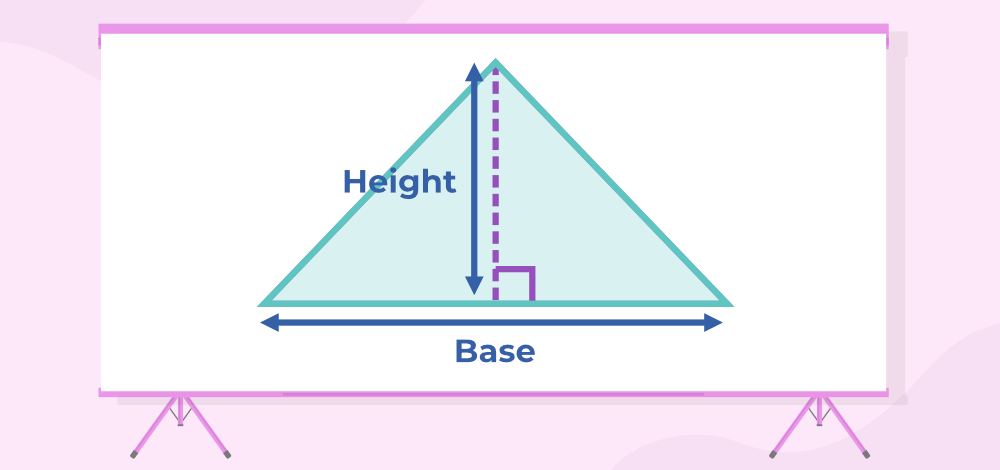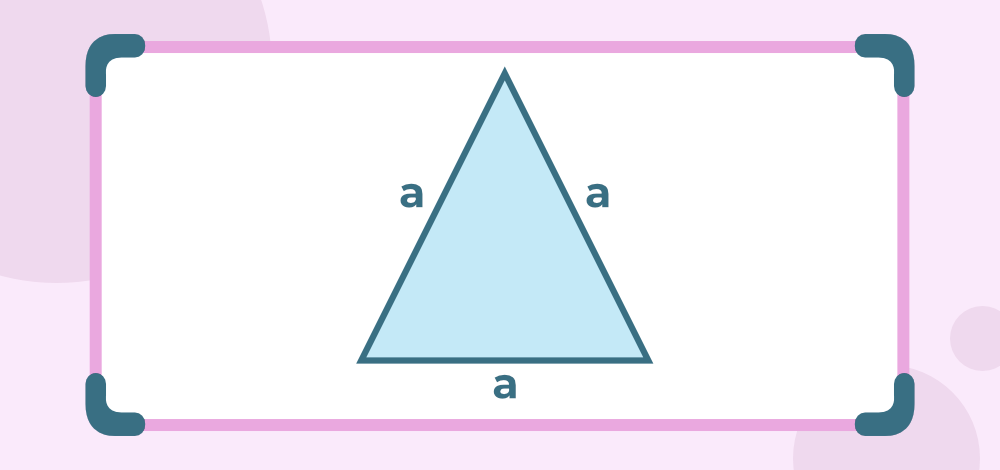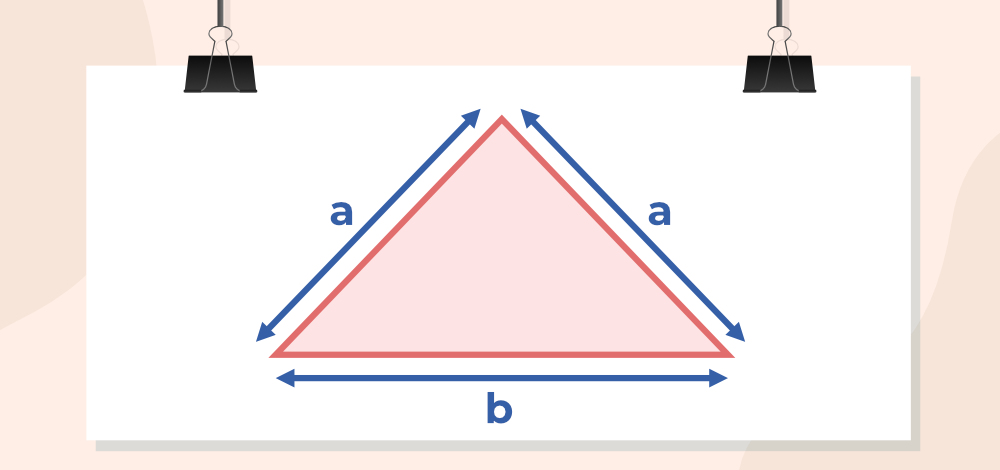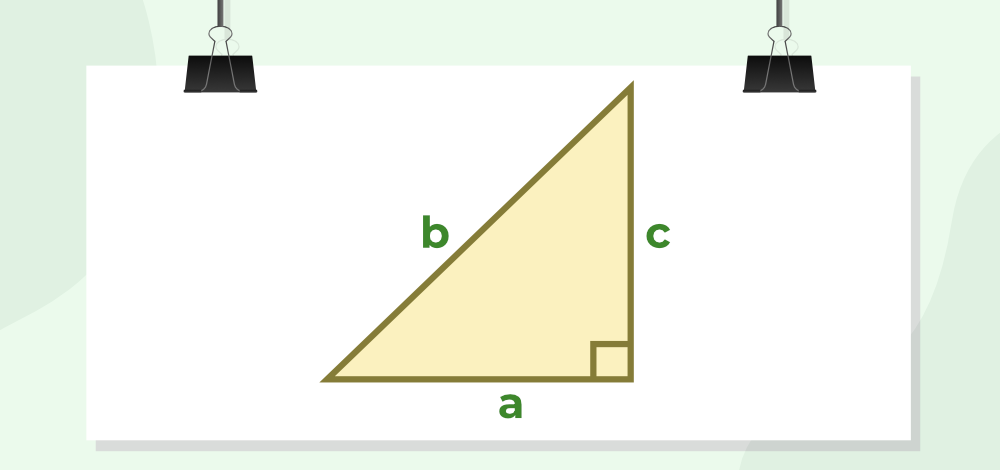GFG App
Open AppBrowser
Continue

Area of a triangle is the region enclosed by these three sides of the triangle i.e. it can be considered as the space occupied by the boundaries of the triangle. We can calculate the area of the triangle if the base and height of the triangle are known. Hence, the area of the triangle can be calculated by the formula half of the product of Base and Height.

Area of the triangle is measured in square units and the SI unit of area is sq. m or m2. Let’s learn about the Area of the Triangle, its various formulas, and other in detail in this article.

## What is the Area of a Triangle?

Area of a triangle is defined as the total surface occupied or enclosed by the boundaries of the triangle on a plane surface. And, the formula for the calculation of the area of a triangle is given by half of the product of the base and height of the given triangle.

Note: Base and the height of a triangle are always perpendicular to each other making a right angle(90 degrees).

### Area of Triangle Definition:

Triangle is the simplest polygon with only three sides. It is a closed figure, which can be Regular or Irregular. A regular triangle is called an Equilateral triangle. On the basis of the sides and angles of triangles, it can be categorized into various categories.

## How to Find the Area of a Triangle?

Area of the triangle is calculated by using the following steps:

Step 1: Mark the dimensions (base, and height) of the given article.

Step 2: Use the formula for finding the area of a triangle. Area = 1/2 × (base × height)

Step 3: The area obtained in step 2 is the required area it is measured in unit2.

Thus, the area of a triangle is found using the above steps.

## Area of Triangle Formula

Area of the triangle depends on the dimensions of the triangle, generally, the area depends on the base and height of the figure. For a triangle, the area is calculated by multiplying half the base by its height. The area of a triangle is measured in square units i.e. m2, cm2, etc.Mathematically the formula is given by:

Area of the triangle(A) = 1/2 × b × h

where,
b is base of the triangle
h is height of the triangle

### Example: What is the area of a triangle with base b = 5 m and height h = 6 m?

Solution:

Using the  general formula, Area of a Triangle, A = 1/2 × b × h

Area = 1/2 × 5 (m) × 6 (m)

= 5 (m) × 3 (m)

= 15 m2

## Area of Triangle when 3 Sides are Given (Heron’s Formula)

Area of a triangle with all three sides given can be found using Heron’s Formula. The application of Herons Formula includes two steps which are discussed below:

Let a triangle with sides a, b, and c are given then for finding its area,Step 1: Calculate the semi-perimeter (s) of the given triangle by

s = (a+b+c)/2

Step 2: Use Heron’s formula and find the required area.

Area = √{s(s-a)(s-b)(s-c)}

where,
s is semiperimeter
a, b, and c are sides of the given triangle.

If in a triangle all three sides are given applying the general formula is very difficult as finding height in such a case is very tricky. So we rely on Heron’s formula in such cases to find the required area.

### Example: What is the area of a triangle with sides of 3 cm, 4 cm, and 5 cm?

Solution:

Using Heron’s formula,

s = (a+b+c)/2

= (3+4+5)/2

= 12/2 = 6

Area = √{ s(s-a)(s-b)(s-c)}

= √{ 6(6-3)(6-4)(6-5)}

= √(6×3×2×1) = √(36)

= 6 cm2

Area of various types of triangles is further discussed in this article.

## Area of Triangle When Given Two Sides and Included Angle (SAS)

For finding the area of a triangle if the two sides of a triangle and an angle included between them are given, use the formula discussed below.

Let us take a triangle PQR, whose vertex angles are ∠P, ∠Q, and ∠R, and the sides are p,q, and r.

Now, when two sides of a triangle and the angle between them are given, then the formulas to calculate the area of a triangle is

• Area (∆PQR) = ½ qr sin P
• Area (∆PQR) = ½ pq sin R
• Area (∆PQR) = ½ rp sin Q

### Example: Find the area of triangle ∆PQR, if P = 60° and q = 3, r = 2 in units.

Solution:

Given, P = 60° and q = 3, r = 2

Area (∆PQR) = ½ qr sin A

= ½ (3) (2) sin 30 (sin 60° = √3/2 )

= 3 × √3/2

=  (3√3) /2 sq.unit.

## Area of an Equilateral Triangle

An equilateral triangle is a triangle having all three sides equal and all three angles equal measuring 60 degrees. Suppose an equilateral triangle of sides a unit is given then the area of an equilateral triangle is given by,Area of an Equilateral Triangle (A) = (√3)/4 × side

= (√3)/4 × a2

### Example: Find the area of a triangle having side a = 8 cm

Solution:

Given,

side of the triangle (a) = 8cm

Area(A) = (√3)/4 × a2

= (√3)/4 × 82

= 16√3 cm2

## Area of an Isosceles Triangle

An isosceles triangle is a triangle having two equal sides and the angles opposite these equal sides are also equal. Suppose an isosceles triangle of common side b and another side of a, then its area is given by,Area of Isosceles triangle = ¼×b√(4a2 – b2)

### Example: Find the area of an isosceles triangle having a common side a = 5 cm and other side b = 8 cm

Solution:

Given,

common side of the triangle (a) = 5cm

other side oftriangle (b) = 8cm

Area(A) = ¼×b√(4a2 – b2)

=  ¼×8√(4×52 – 82)

=  2×6 = 12 cm2

Area of Isosceles Triangle

## Area of a Right Angled Triangle

A triangle that contains a right angle is considered a right-angled triangle. If all three sides in the right-angled triangle are given then applying the general formula then Heron’s formula is very easy. As one of the sides in the right-angle triangle behaves as the base and the other as height. In the figure given below, a is the base of the triangle, and c is its height of it. So applying the general formula is easy here,Area of the triangle(A) = 1/2 × a × c

where,
a is base of the triangle
c is height of the triangle

### Example: Find the area of a triangle having a base of a = 8 cm and a height c = 5 cm.

Solution:

Given

Base of the triangle (a) = 8cm

Height of the triangle (c) = 5cm

We have,

Area(A) = 1/2 × a × c

= 1/2 × 8 × 5

= 20 cm2

## What is the Perimeter of a Triangle?

Perimeter of the triangle is defined as the total length of the boundary of the triangle. For example, if a triangle has three sides of length a m, b m, and c m then its perimeter is (a + b + c) m.

Let a triangle of the triangle ABC is taken then,

Perimeter of △ABC = AB + BC + CA

### Example: The triangle park has three sides of 15 m, 18 m, and 20 m. If a man runs along the boundary of the park 5 times find the distance travelled by him.

The side of the park is,

• a = 15 m
• b = 18 m
• c = 20 m

Perimeter of the Park = a + b + c

= 15 + 18 + 20 = 53 m

The distance travelled by the man = 5 × 53

= 165 m

Related Articles

## Solved Examples on Area of a Triangle

### Example 1: Find the area of a triangle having a base of 12 cm and a height of 4 cm.

Solution:

Given

Base of the triangle = 12cm’

Height of the triangle = 4cm

We have,

Area(A) = 1/2 × b × h

= 1/2 × 12 × 4

= 24cm2

### Example 2: What is the area of a triangle with sides of 8 cm, 6 cm, and 10 cm (using Heron’s Formula)?

Solution:

Using Heron’s formula,

s = (a+b+c)/2

= (8+6+10)/2

= 24/2 = 12

Area = √{ s(s-a)(s-b)(s-c)}

= √{ 12(12-8)(12-6)(12-10)}

= √(12×4×6×2) = √(576)

= 24 cm2

### Example 3: Find the area of a right-angled triangle having base a = 5 cm and height c = 3 cm.

Solution:

Given

Base of the triangle (a) = 5 cm

Height of the triangle (c) = 3 cm

We have,

Area(A) = 1/2 × a × c

= 1/2 × 5 × 3

= 7.5 cm2

### Example 4: Find the area of an equilateral triangle having side a = 6 cm

Solution:

Given,

side of the triangle (a) = 6 cm

Area(A) = (√3)/4 × a2

= (√3)/4 × 62

= 9√3 cm2

### Example 5: Find the area of a triangle having a base of 15cm and a height of 5cm.

Solution:

The base of the triangle=15 cm

Height of the triangle=5 cm

We have,

Area(A) = 1/2 × b × h

= 1/2 × 15 × 5

= 37.5 cm2

## FAQs on Area of Triangle

### Question 1: What is the Area of the Triangle?

The region enclosed by the boundary of the triangle i.e. area occupied by the perimeter of the triangle is termed the area of the Triangle.

### Question 2: How to calculate the area of a triangle?

Area of the Triangle can be calculated using the formula,

Area = 1/2 × b × h

where
b is the base of triangle
h is the height of triangle

### Question 3: What is an area of a triangle when vertices are given?

When all three vertices of the triangle A(x1, y1), B(x2, y2) and C(x3, y3) are given then its area is calculated using the formula,

Area = 1/2 × [x1(y2 – y3) + x2(y3 – y1) + x3(y1 – y2)]

### Question 4: What is the area of a triangle when three sides are given?

If all the three sides of Triangle are given then its area is calculated using the Herons Formula. Let the side of triangle be a, b, and c then its area is

Area = √{ s(s-a)(s-b)(s-c)}

where,
s is semi-perimeter

### Question 5: What is an area triangle in vector form?

Area = 1/2|×|